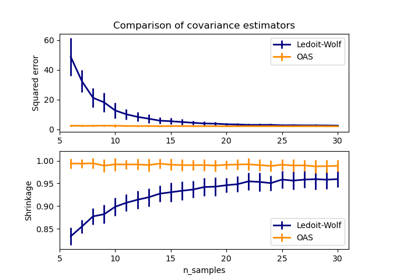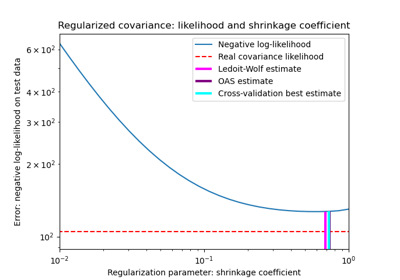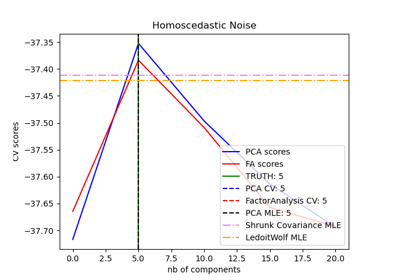# sklearn.covariance.LedoitWolf¶

class sklearn.covariance.LedoitWolf(*, store_precision=True, assume_centered=False, block_size=1000)[source]

LedoitWolf Estimator.

Ledoit-Wolf is a particular form of shrinkage, where the shrinkage coefficient is computed using O. Ledoit and M. Wolf’s formula as described in “A Well-Conditioned Estimator for Large-Dimensional Covariance Matrices”, Ledoit and Wolf, Journal of Multivariate Analysis, Volume 88, Issue 2, February 2004, pages 365-411.

Read more in the User Guide.

Parameters:
store_precisionbool, default=True

Specify if the estimated precision is stored.

assume_centeredbool, default=False

If True, data will not be centered before computation. Useful when working with data whose mean is almost, but not exactly zero. If False (default), data will be centered before computation.

block_sizeint, default=1000

Size of blocks into which the covariance matrix will be split during its Ledoit-Wolf estimation. This is purely a memory optimization and does not affect results.

Attributes:
covariance_ndarray of shape (n_features, n_features)

Estimated covariance matrix.

location_ndarray of shape (n_features,)

Estimated location, i.e. the estimated mean.

precision_ndarray of shape (n_features, n_features)

Estimated pseudo inverse matrix. (stored only if store_precision is True)

shrinkage_float

Coefficient in the convex combination used for the computation of the shrunk estimate. Range is [0, 1].

n_features_in_int

Number of features seen during fit.

New in version 0.24.

feature_names_in_ndarray of shape (n_features_in_,)

Names of features seen during fit. Defined only when X has feature names that are all strings.

New in version 1.0.

EllipticEnvelope

An object for detecting outliers in a Gaussian distributed dataset.

EmpiricalCovariance

Maximum likelihood covariance estimator.

GraphicalLasso

Sparse inverse covariance estimation with an l1-penalized estimator.

GraphicalLassoCV

Sparse inverse covariance with cross-validated choice of the l1 penalty.

MinCovDet

Minimum Covariance Determinant (robust estimator of covariance).

OAS

Oracle Approximating Shrinkage Estimator.

ShrunkCovariance

Covariance estimator with shrinkage.

Notes

The regularised covariance is:

(1 - shrinkage) * cov + shrinkage * mu * np.identity(n_features)

where mu = trace(cov) / n_features and shrinkage is given by the Ledoit and Wolf formula (see References)

References

“A Well-Conditioned Estimator for Large-Dimensional Covariance Matrices”, Ledoit and Wolf, Journal of Multivariate Analysis, Volume 88, Issue 2, February 2004, pages 365-411.

Examples

>>> import numpy as np
>>> from sklearn.covariance import LedoitWolf
>>> real_cov = np.array([[.4, .2],
...                      [.2, .8]])
>>> np.random.seed(0)
>>> X = np.random.multivariate_normal(mean=[0, 0],
...                                   cov=real_cov,
...                                   size=50)
>>> cov = LedoitWolf().fit(X)
>>> cov.covariance_
array([[0.4406..., 0.1616...],
[0.1616..., 0.8022...]])
>>> cov.location_
array([ 0.0595... , -0.0075...])


Methods

 error_norm(comp_cov[, norm, scaling, squared]) Compute the Mean Squared Error between two covariance estimators. fit(X[, y]) Fit the Ledoit-Wolf shrunk covariance model to X. get_params([deep]) Get parameters for this estimator. Getter for the precision matrix. Compute the squared Mahalanobis distances of given observations. score(X_test[, y]) Compute the log-likelihood of X_test under the estimated Gaussian model. set_params(**params) Set the parameters of this estimator.
error_norm(comp_cov, norm='frobenius', scaling=True, squared=True)[source]

Compute the Mean Squared Error between two covariance estimators.

Parameters:
comp_covarray-like of shape (n_features, n_features)

The covariance to compare with.

norm{“frobenius”, “spectral”}, default=”frobenius”

The type of norm used to compute the error. Available error types: - ‘frobenius’ (default): sqrt(tr(A^t.A)) - ‘spectral’: sqrt(max(eigenvalues(A^t.A)) where A is the error (comp_cov - self.covariance_).

scalingbool, default=True

If True (default), the squared error norm is divided by n_features. If False, the squared error norm is not rescaled.

squaredbool, default=True

Whether to compute the squared error norm or the error norm. If True (default), the squared error norm is returned. If False, the error norm is returned.

Returns:
resultfloat

The Mean Squared Error (in the sense of the Frobenius norm) between self and comp_cov covariance estimators.

fit(X, y=None)[source]

Fit the Ledoit-Wolf shrunk covariance model to X.

Parameters:
Xarray-like of shape (n_samples, n_features)

Training data, where n_samples is the number of samples and n_features is the number of features.

yIgnored

Not used, present for API consistency by convention.

Returns:
selfobject

Returns the instance itself.

get_params(deep=True)[source]

Get parameters for this estimator.

Parameters:
deepbool, default=True

If True, will return the parameters for this estimator and contained subobjects that are estimators.

Returns:
paramsdict

Parameter names mapped to their values.

get_precision()[source]

Getter for the precision matrix.

Returns:
precision_array-like of shape (n_features, n_features)

The precision matrix associated to the current covariance object.

mahalanobis(X)[source]

Compute the squared Mahalanobis distances of given observations.

Parameters:
Xarray-like of shape (n_samples, n_features)

The observations, the Mahalanobis distances of the which we compute. Observations are assumed to be drawn from the same distribution than the data used in fit.

Returns:
distndarray of shape (n_samples,)

Squared Mahalanobis distances of the observations.

score(X_test, y=None)[source]

Compute the log-likelihood of X_test under the estimated Gaussian model.

The Gaussian model is defined by its mean and covariance matrix which are represented respectively by self.location_ and self.covariance_.

Parameters:
X_testarray-like of shape (n_samples, n_features)

Test data of which we compute the likelihood, where n_samples is the number of samples and n_features is the number of features. X_test is assumed to be drawn from the same distribution than the data used in fit (including centering).

yIgnored

Not used, present for API consistency by convention.

Returns:
resfloat

The log-likelihood of X_test with self.location_ and self.covariance_ as estimators of the Gaussian model mean and covariance matrix respectively.

set_params(**params)[source]

Set the parameters of this estimator.

The method works on simple estimators as well as on nested objects (such as Pipeline). The latter have parameters of the form <component>__<parameter> so that it’s possible to update each component of a nested object.

Parameters:
**paramsdict

Estimator parameters.

Returns:
selfestimator instance

Estimator instance.

## Examples using sklearn.covariance.LedoitWolf¶Ledoit-Wolf vs OAS estimation

Ledoit-Wolf vs OAS estimationShrinkage covariance estimation: LedoitWolf vs OAS and max-likelihood

Shrinkage covariance estimation: LedoitWolf vs OAS and max-likelihoodModel selection with Probabilistic PCA and Factor Analysis (FA)

Model selection with Probabilistic PCA and Factor Analysis (FA)